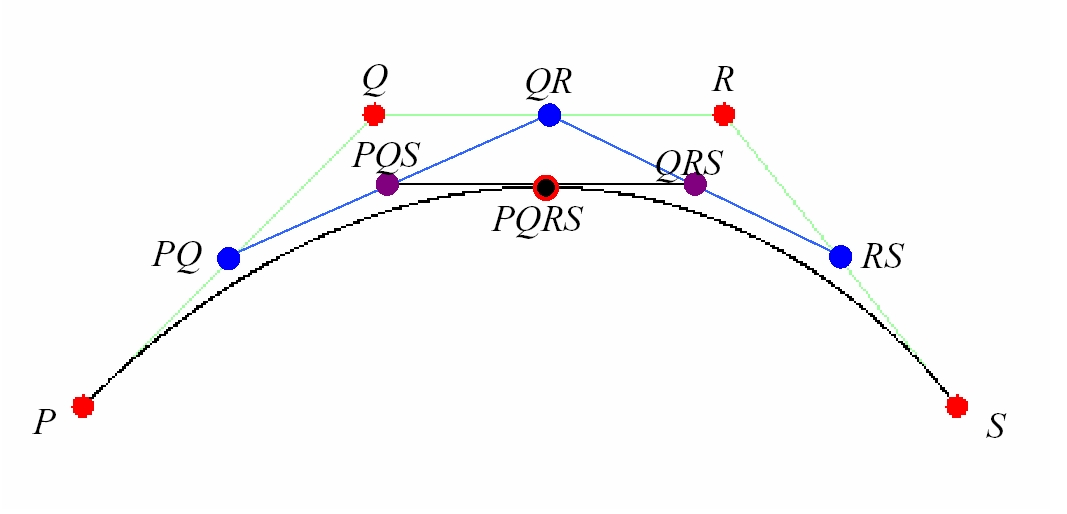Bezier Cubics

 A cubic Bezier curve is determined by 4 control points P, Q, R, S, shown in red. The curve starts at the point P and ends at the point S. Its tangent at P is parallel to the line segment PQ and its tangent at S is parallel to the line segment RS. It does not generally pass through the points Q or R. It is always contained within the convex hull of the four control points. The parametric form of the curve is B(t) = (1 - t)3 + 3(1 - t)2t + 3t2(1 - t) + t3 In this applet, the curve is generated recursively as follows. Compute the midpoints of each of the line segments shown. PQ = (P + Q)/2 QR = (Q + R)/2 RS = (R + S)/2 Compute the midpoints of the two line segments PQ to QR and QR to RS. PQR = (PQ + QR)/2 QRS = (QR + RS)/2 Compute the midpoint of the line segment PQR to QRS PQRS = (PQR + QRS)/2 Then B(1/2) = PQRS and the Bexier cubic determined by P, Q, R, and S is the union of the Bezier cubic determined by P, PQ, PQR, and PQRS and the Bezier cubic determined by PQRS, QRS, RS, S.source: bezier extends BufferedApplet (Ken Perlin) Click and drag the control points to change the curve.